# Why capacitive reactance reduces inductive reactance (by intuition/physically not mathematically)?

#### simon88

Joined Dec 2, 2022
6
Hi I am newbie here! I understand why capacitive reactance reduces inductive reactance mathematically, having a result of zero at resonant frequency. But how to physically explain the flow of electrons in a series LC circuit, where the coil lags the flow of current (electrons) relative to the applied voltage. How can the capacitor counteract this? By reducing the coil's back EMF? I assume, that if coil blocks electrons flow (current), capacitor cant counteract it, especially when there is no voltage on its terminals. As the voltage of the power source increases, the first thing it encounters is a coil that prevents it from flowing current. How can the capacitor counteract this, if from the point of view of the power source it is a short circuit at the very beginning? Thank in advance for any explanation.

#### BobTPH

Joined Jun 5, 2013
6,302
It does not counteract the inductance. The current still lags the voltage in the inductor in the resonant circuit in the same way. What it does is gives a place for the energy to go such that none is lost, it just flows back and forth between the current in the inductor and the charge in the capacitor.

#### MrSalts

Joined Apr 2, 2020
2,767
none is lost
In an "ideal" inductor and ideal capacitor, of course.

#### simon88

Joined Dec 2, 2022
6
We say that at resonance frequency voltage and current are in phase. However, If coil still causes that current lags the voltage, there should my no current in circuit when voltage source is increasing. Current in every point in circuit should be the same (Kirchhoff's law). So on the left and right side of both coil and capacitor curent also can't flow due to the coil's reactance. Am I wrong?

Of course we are talking about ideal LC circuit.

#### ericgibbs

Joined Jan 29, 2010
17,170
hi Simon,
Perhaps a visual representation would make it clearer.
E
Two plots , one with an ideal 0R inductor [ Undamped ] and one with an inductor having a 1R internal resistance.
The Initial Condition is that Vi, .IC = 100V , then instantly removed.
E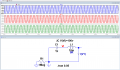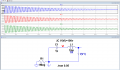#### simon88

Joined Dec 2, 2022
6
Thank you Eric. I found very useful animation made by Eugene Khutoryansky on YouTube (search: Resonance Circuits: LC Inductor-Capacitor Resonating Circuits). However i wonder where is coil's back EMF in this circuit (on video)? It looks like there is no opposite force in coil due to current, which comes from capacitor (when capacitor is discharching)?

#### ericgibbs

Joined Jan 29, 2010
17,170
hi Simon.
For idealized components, the capacitor and inductor exchange their energy.
As you may know that for a Capacitor the Current leads the Voltage and in an Inductor the Voltage leads the Current.

So in a resonant Series Cap and Inductor circuit, the currents through the two components are 180 deg out of phase.

So the components simply keep exchanging their stored energy.
E

#### MrSalts

Joined Apr 2, 2020
2,767
We say that at resonance frequency voltage and current are in phase. However, If coil still causes that current lags the voltage, there should my no current in circuit when voltage source is increasing. Current in every point in circuit should be the same (Kirchhoff's law). So on the left and right side of both coil and capacitor curent also can't flow due to the coil's reactance. Am I wrong?

Of course we are talking about ideal LC circuit.
Kirchoff's applies but instantaneously, you can't use RMS voltages.

#### crutschow

Joined Mar 14, 2008
31,533
Here is another simulation of an ideal LC resonant circuit;

Note that the peak voltage is reached when the inductor current is zero, indicating all the inductor energy has been transferred to the capacitor.
Similarly when the maximum inductor current is reached, the voltage is zero, indicating all the capacitor energy has been transferred to the inductor.
Thus, in a resonant circuit, the energy is transferred back and forth between the inductor and the capacitor, which causes the voltage and current to be 90° out of phase.
Where the two waveforms cross, half the energy has been transferred from one to the other.

As an exercise, if you calculate the capacitor energy at the peak voltage, and the inductor energy at the peak current, you will see they are equal.

We normally don't talk about "back EMF" in a resonant circuit as that really has no meaning.
Inductive back EMF occurs when you try to suddenly stop the current through an inductor.
But the inductive EMF here is what generates the voltage that charges the capacitor until its inductive energy is zero.
Then the capacitor voltage increases the current through the inductor to transfer its energy back to the inductor.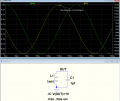Last edited:
•nsaspook

#### WBahn

Joined Mar 31, 2012
28,182
Hi I am newbie here! I understand why capacitive reactance reduces inductive reactance mathematically, having a result of zero at resonant frequency. But how to physically explain the flow of electrons in a series LC circuit, where the coil lags the flow of current (electrons) relative to the applied voltage. How can the capacitor counteract this? By reducing the coil's back EMF? I assume, that if coil blocks electrons flow (current), capacitor cant counteract it, especially when there is no voltage on its terminals. As the voltage of the power source increases, the first thing it encounters is a coil that prevents it from flowing current. How can the capacitor counteract this, if from the point of view of the power source it is a short circuit at the very beginning? Thank in advance for any explanation.
By "at the very beginning", are you talking about when the AC power is first applied after the circuit has been sitting there with 0 V across it long enough without power so that that there is no current and no voltage across the capacitor?

If so, then you are talking about the transient response period and we can't talk about the circuit as though it is in AC steady state.

Consider the following circuit: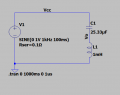The sinusoidal source is set to not start for the first 100 ms so that the circuit's initial conditions will be for everything de-energized. There is a 0.1 Ω series resistance to keep the response well bounded. Without it, the response is largely dictated by simulation artifacts.

Here is the overall simulation, zoomed in to show the transient period.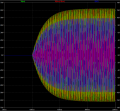As we can see, it takes about 100 ms to reach AC stead state (the amount of time taken to achieve steady state is predominantly determined by the series resistance for fixed L and C).

If we zoom in on the first few cycles after the AC source starts:We see that the voltages across the capacitor (red) and inductor (green) are NOT 180° out of phase, nor are they in quadrature (90°) relationships with the current (blue). This is because things are changing and the circuit is not, yet, in AC steady state. It is the fact that the relationships are not phase-locked that allows the energy that is eventually shared by the capacitor and inductor (which will be shuttled back and forth between the two in steady state) to be pumped into them from the source during the transient period of operation.

Although the overall voltage (Vcc) isn't plotted, we know that it peaks every 1 ms. Note that the current is NOT at zero (i.e., the voltage and the current of the source are also not in quadrature). This is because there is net energy flowing from the source to the circuit during the transient, which is being transferred to the L and the C.

If we shift down the time axis a half a second to when the circuit IS in AC steady state:We see the relationships we expect. IN particular, the voltages across the L and the C are equal and opposite at all times and the current is in quadrature with the supply voltage.

#### WBahn

Joined Mar 31, 2012
28,182
hi Simon.
For idealized components, the capacitor and inductor exchange their energy.
As you may know that for a Capacitor the Current leads the Voltage and in an Inductor the Voltage leads the Current.

So in a resonant Series Cap and Inductor circuit, the currents through the two components are 180 deg out of phase.

So the components simply keep exchanging their stored energy.
E
How can the statement in bold be true if the two components are in series?

Because they are in series, the currents through the two MUST be in phase (they are the same current!).

But the voltage across the capacitor lags this current by 90° while the voltage across the inductor leads this current by 90°, which means that the voltage across the two components is 180° out of phase.

#### schmitt trigger

Joined Jul 12, 2010
515
If you think of the mechanical analogous, a mass and a spring, it is significantly easier to visualize.

#### simon88

Joined Dec 2, 2022
6
We see that the voltages across the capacitor (red) and inductor (green) are NOT 180° out of phase, nor are they in quadrature (90°) relationships with the current (blue). This is because things are changing and the circuit is not, yet, in AC steady state. It is the fact that the relationships are not phase-locked that allows the energy that is eventually shared by the capacitor and inductor (which will be shuttled back and forth between the two in steady state) to be pumped into them from the source during the transient period of operation.

Although the overall voltage (Vcc) isn't plotted, we know that it peaks every 1 ms. Note that the current is NOT at zero (i.e., the voltage and the current of the source are also not in quadrature). This is because there is net energy flowing from the source to the circuit during the transient, which is being transferred to the L and the C.
I think i start to get it slowly. I have additional question. Let's say we have steady state in series LC circuit with AC source and capacitor is fully charged (maximum Vc) and for less than millisecond no current is flowing. We see that at the same time coil's voltage is at its peak value, but across the the coil and capacitor voltages are 180° out of phase. So they both (coil and capacitor) behave a little like two identical batteries connected in series but in opposite direction (one blocks the second one). Is coils high voltage at this particular moment a result of inductive EMF? Does it mean that coil's voltage opposite direction tries to prevent dischring by capacitor?

#### BobTPH

Joined Jun 5, 2013
6,302
It is a result of the inductor equation:

V = L dI / dt

#### crutschow

Joined Mar 14, 2008
31,533
capacitor is fully charged (maximum Vc) and for less than millisecond no current is flowing
Current is always flowing, either into or out of the capacitor.
There is an infinitesimal short time when it goes from charging to discharging during the oscillation.
Does it mean that coil's voltage opposite direction tries to prevent dischring by capacitor?
If I understand you correctly, yes.
The inductor's inductance resists any change in current (similar to the inertia of a mass) by generating a voltage across it, according to the equation posted by BobTPH.

#### WBahn

Joined Mar 31, 2012
28,182
I think i start to get it slowly. I have additional question. Let's say we have steady state in series LC circuit with AC source and capacitor is fully charged (maximum Vc) and for less than millisecond no current is flowing. We see that at the same time coil's voltage is at its peak value, but across the the coil and capacitor voltages are 180° out of phase. So they both (coil and capacitor) behave a little like two identical batteries connected in series but in opposite direction (one blocks the second one). Is coils high voltage at this particular moment a result of inductive EMF? Does it mean that coil's voltage opposite direction tries to prevent dischring by capacitor?
If it is AC steady state, then the moments when no current is flowing are just that -- instantaneous moments.

But let's assume that, somehow, that no current is flowing for some period of time. That means that the current is not changing during that period of time, which therefore means that there is no voltage across the inductor, since voltage across an inductor is proportional to the rate at which the current is changing.

But that's not what is happening in AC steady state. In that case, the current through both components is changing sinusoidally. When the current is zero is precisely when it is changing the fastest. For the capacitor, this happens at the moment of maximum change and, hence, maximum voltage. For the inductor, the fact that it is changing the fastest means that it is also the moment of maximum voltage across it. This is also the point at which all of the stored energy is in the capacitor and none of it is in the inductor.

Similarly, when the voltage across the capacitor is zero, this is the moment of maximum current, meaning that none of the energy is in the capacitor and it all is in the inductor. This is also the moment that the change of current is zero, just as the current transitions from increasing to decreasing, and hence there is no voltage across the inductor, either.

#### simon88

Joined Dec 2, 2022
6
Ok, using the example above, here is my understanding od LC series circuit with AC power source.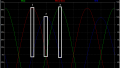(1) Capacitor is fully charged and starts do discharge with its maximum Vc. A the same time coils voltage has the same voltage but in opposite direction, because it wants to prevent current flow. However the capacitor starts discharging slowly. The currents starts to flow.
(2) Capacitor is fully discharged. However coil doesn't like change in current, so it creates a voltage whose polarity is in the direction of current flow. I imagine, that at resonance frequency this is exactly the moment, when AC source voltage starts to increase at the same direction 'helping' coil to push current toward second plate of the capacitor?
(3) The same situation like in point (1), but in opposite direction.

What is a reason that coils voltage increase between (2) and (3)? Am I right that it is related to te decreasing current, which coil wants to prevent (inductive EMF?)?

P.S. Sorry If sometimes I am not precisely speaking. English is not my native language, as you suppose#### ericgibbs

Joined Jan 29, 2010
17,170
Hi Simon.
Do you know how the inductor 'stores' the energy and why it 'resists' the current build up.?
E